# Program to check Sunny Number in Java

In this tutorial you will learn about the Program to check Sunny Number in Java and its application with practical example.

In this tutorial, we will learn to create a Java Program to check Sunny Numbers in Java using Java programming.

## Prerequisites

Before starting with this tutorial we assume that you are best aware of the following Java programming topics:

• Java Operators.
• Basic Input and Output function in Java.
• Class and Object in Java.
• Basic Java programming.
• If-else statements in Java.
• For loop in Java.

What is Sunny Number.

Sunny number is a number,if  next  number to a given number is a perfect square.

For Example=> 80 is a Sunny Number because 81 is a perfect square of 9.

## Program to check Sunny Number in Java

In this program we would find given number is a Sunny number or not .first of we would take a value from user and find the Sunny number.let have a look at the code.

## Output

Sunny Number.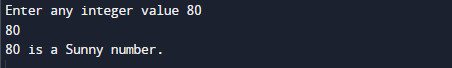Not a Sunny Number.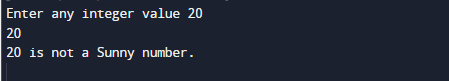In the above program, we have first declared and initialized a set variables required in the program.

• num,numberand= it will hold entered number.
• result   it will hold result it means number is a sunny or not.

After that we take a number from user and find given number is a Sunny number or not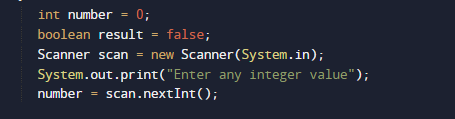and pass this  number to Sunny_no() function where we check condition for sunny number or we cans say condition for Perfect square where we add one to the number to check it is Sunny Number or not.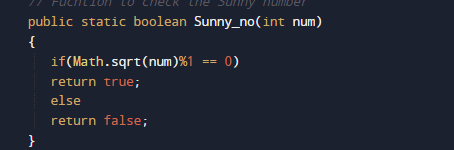Function return value true if number is Perfect square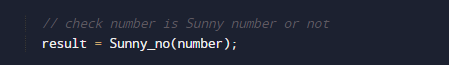else return false and we will store the return value in variable result.if value of result is true number is Sunny and if value of result if false number is not a Sunny number.

In this tutorial we have learn about the Program to check Sunny Number in Java and its application with practical example. I hope you will like this tutorial.